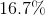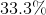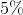# PSAT Math : Percentage

## Example Questions

### Example Question #2 : How To Find Amount Of Profit

Ricky works at a shoe shop, and earns $40 in commission for each pair of shoes he sells plus a$100 weekly salary. If Ricky receives no other money, which of the following expressions represents the total dollar amount Ricky receives for a week in which he sells n shoes?

140n

140 + n

100 + 40n

100 + n

40(100 + n)

100 + 40n

Explanation:

If Ricky sells n shoes in a week, he earns $40n in commission. His salary is a constant$100 per week, so his total payout is $100 +$40n.

### Example Question #1 : How To Find Amount Of Profit

An entrepreneur started a company making floggles.  The factory requires $1000 worth of fixed expenses to keep it running every month. She is able to produce one floggle at the cost of$4 and sell one floggle at the cost of $6. If she produces and sells 500 floggles in one month, what is her profit? Possible Answers:$1000

$2000$0

$3000$4000

$0 Explanation: Profit = Income - Expenditures Income =$6/floggle times 500 floggles = $3000 Expenditures =$1000 + $4/floggle times 500 floggles =$1000 + $2000 =$3000

Profit = 3000 - 3000 = 0

### Example Question #1 : Profit Margin

You are planning a New Year’s Eve bash.  For each person attending, the caterer will charge you $15 for food,$10 for beverages, $5 for service. The band charges$2000 for the entire evening.  You also have to pay the venue $2500 to rent the location for the night and$3 for parking for each attendee.  If you expect 500 people to attend and you would like to make a $10000 profit for planning the event, how much must each ticket cost? Possible Answers:$62

$53$42

$33$60

$62 Explanation: First determine total cost. Caterer: Per person =$15 + $10 +$5 = $30 per person Parking: Per person =$3 per person

Total per person = $33$33 * 500 people = $16,500 Plus cost of renting venue + band =$2500 + $2000 =$4500

Total (net) cost =  $16,500 +$4500  = $21,000 Total (gross) cost = net cost + profit =$21,000 + $10,000 =$31,000

Cost per ticket = Gross cost / # of attendee = $31,000 / 500 =$62

### Example Question #1 : How To Find Amount Of Profit

The Widget Company has annual revenues of $150,000. Their expenses over the same time frame was$75,000.  What was the percent profit?

30%

25%

60%

75%

50%

50%

Explanation:

Profit = Revenue – Expense

% Profit = $Profit ÷$ Total Revenue

% Profit = ($150,000 –$75,000) ÷ $150,000 = 50% ### Example Question #1 : How To Find Amount Of Profit Nicki sold 20 albums at$5 each. How many albums should Minaj sell at $4.50 to earn more than Nicki? Possible Answers: 23 22 24 19 25 Correct answer: 23 Explanation: The answer is 23. 23*$4.50 = $103.50, which is more than what Nicki earned. ### Example Question #4 : How To Find Amount Of Profit During Laura and Anna’s bake sale, 35 brownies, 12 cupcakes and 23 glasses of lemonade were sold. These goods cost$44 for the raw ingredients, and they sold for $79. What is the average profit per item? Possible Answers:$1.75

$0.25$0.35

$0.70$0.50

$0.50 Explanation: Total profit ($35) divided by total items (70) yields the answer of $0.50 profit per item. ### Example Question #171 : Percentage Each wooden chair that a carpenter makes requires$20 worth of supplies. He then sells the chairs for $50 each. The carpenter recently discovered a new supplier that would allow him to spend 25% less on supplies. If he doesn't change his selling price, by what percent could the carpenter increase his profit by using the new supplier? Possible Answers:Correct answer:Explanation: Using$20 worth of supplies and selling the chairs for $50 each, the carpenter is originally making a profit of$30 per chair.

The new supplier would reduce costs by 25% or 1/4. One-fourth of $20 is$5, so the new supplier would be $5 less, or$15.

### Example Question #1 : How To Find The Amount Of Sales Tax

If there is a 10% sale on an item, and then 9% sales tax is applied to that after-sale price, then what is the total cost of the item including tax as a percentage of its pre-sale sticker price?

101%

81.9%

99.2%

98.1%

99%

98.1%

Explanation:

A 10% sale means that the post-sale price of the item is now 90%, or 0.9 of the original cost of the item. We then apply 9% sales tax by multiplying the 0.9 by 109%, or 1.09. 0.9 * 1.09 = .981, so the total cost of the item is 98.1% of the original pre-sale sticker price.

For percentage problems that do not deal with a specific starting number, it is always helpful to plug in 100 for the starting number. Here, we would then have a post-sale price of 90 dollars, and if we calculate the sales tax for the 90-dollar item it would be 90 * 0.09 = $8.10. THis gives us a total cost of 90 + 8.10 =$98.10, or 98.1% of the original 100-dollar price.

### All PSAT Math Resources